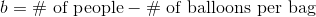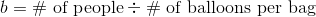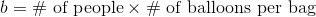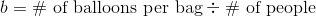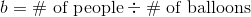# ISEE Lower Level Math : How to divide

## Example Questions

← Previous 1 3 4 5 6 7

### Example Question #1 : How To Divide

Which number has a remainder of 3 when divided by 8?

20

17

12

11

18

11

Explanation:

11 ÷ 8 =

1 remainder 3.

20 ÷ 8 =

2 remainder 4.

17 ÷ 8 =

2 remainder 1.

18 ÷ 8 =

2 remainder 2.

12 ÷ 8 =

1 remainder 4.

### Example Question #84 : Operations

Find the quotient, when= 6.  Round to the nearest hundreths place.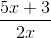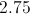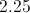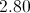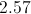Explanation: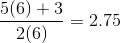### Example Question #3 : How To Divide

Solve the expression.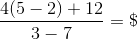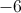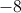Explanation: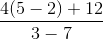We can re-write the expression using division.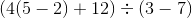We need to use order of operations to solve. First, evaluate the parenthesis.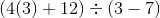In the first parenthesis, we need to multiply before we add.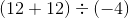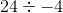Finally, we can divide. Remember that a positive divided by a negative is a negative.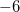### Example Question #85 : Operations

Read the following problem but do not solve it.

Mr. Jones has provided in his will that his 1,790-acre farm will be distributed equally among his five children. How much land will each child inherit?

Which of the following expressions must be evaluated in order to answer this question?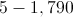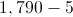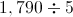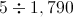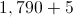Explanation:

"Distributed equally" is a phrase that indicates a division. Divide the number of acres by the number of children: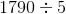is the expression that must be evaluated.

### Example Question #1 : How To Divide

What number is divisible by 7 without a remainder?

24

44

36

28

28

Explanation:

The only number here that can be evenly divided by 7 is 28: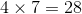### Example Question #1 : How To Divide

What number is divisible by 7 without a remainder?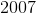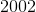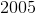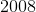Explanation:

First divide any of the answers by 7: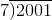Which gives the answer of 285, with a remainder of 6. This is almost 7, so try a number slightly larger: 2002.Which gives an answer of 286, with no remainder.

### Example Question #7 : How To Divide

Which of the following is divisible by 4 (without a remainder)?

4,202

10,334

7,936

12,114

7,936

Explanation:

Because all multiples of 100 are divisible by 4, if the last 2 numbers are divisible by 4, the entire number is divisible by 4.

36 is divisible by 4, while 2, 14, and 34 are not. Therefore, 7,936 is the only number that is divisible by 4.

### Example Question #2 : How To Divide

What is the quotient ofand?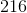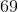Explanation:

A quotient is the result of a division problem.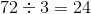Therefore, the quotient ofandis.

### Example Question #9 : How To Divide

Sarah has a box of cookies, and she wants to share them evenly with everyone in her class. She knows how many cookies she has and how many students are in the class. How does she figure out how many cookies,, to give to each student?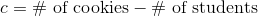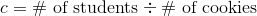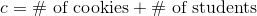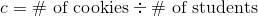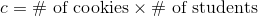Explanation:

When someone is trying to share something equally among a group, it's a division problem. To calculate the cookies per student, divide the item shared (cookies) by the number of students.

### Example Question #10 : How To Divide

Tom is at the store and needs to buy balloons for his upcoming party. He wants to make sure he buys a balloon for each person who comes to the party. How should he decide how many bags,, of balloons to buy?